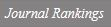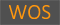The existence of infinitely many solutions for nonlinear elliptic equations involving p-Laplace type operators in R-N

Title
The existence of infinitely many solutions for nonlinear elliptic equations involving p-Laplace type operators in R-N
Authors
Kim, Yun-HoBae, Jung-HyunLee, Jongrak
Ewha Authors
Issue Date
2017
Journal Title
JOURNAL OF NONLINEAR SCIENCES AND APPLICATIONS
ISSN
2008-18982008-1901Citation
JOURNAL OF NONLINEAR SCIENCES AND APPLICATIONS vol. 10, no. 4, pp. 2144 - 2161
Keywords
p-Laplace typeweak solutioniteration methodfountain theorem.
Publisher
INT SCIENTIFIC RESEARCH PUBLICATIONS
Indexed
SCIE; SCOPUSDocument Type
Article
Abstract
We are concerned with the following nonlinear elliptic equations -div (phi(x, del u)) + b (x)vertical bar u vertical bar(p-2) u = lambda f (x, u) in R-N, where the function phi(x, v) is of type vertical bar v vertical bar p(-2)v, b : R-N -> (0, infinity) is a continuous potential function, lambda is a real parameter, and f : R-N x R -> R is a Carath 'eodory function. In this paper, under suitable assumptions, we show the existence of infinitely many weak solutions for the problem above without assuming the Ambrosetti and Rabinowitz condition, by using the fountain theorem. Next, we give a result on the existence of a sequence of solutions for the problem above converging to zero in the L-infinity-norm by employing the Moser iteration under appropriate conditions. (C) 2017 All rights reserved.
DOI
10.22436/jnsa.010.04.67
Appears in Collections:
연구기관 > 수리과학연구소 > Journal papers
Files in This Item:
There are no files associated with this item.
Export
RIS (EndNote)
XLS (Excel)
XML